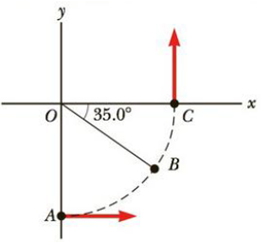Chapter 7, Problem 15P

Chapter
Section
Textbook Problem

A car initially traveling eastward turns north by traveling in a circular path at uniform speed as shown in Figure P7.15. The length of the arc ABC is 235 m, and the car completes the turn in 36.0 s. (a) Determine the car’s speed. (b) What is the magnitude and direction of the acceleration when the car is at point B?Figure P7.15

(a)

To determine
The speed of the car.

Explanation

Given Info: The length of the arc ABC is 235m and the time for the car to traverse the path is 36.0s .

Explanation: The car moves in an arc of a circular path with a constant speed.

The formula for the speed of the car is,

v=ΔsΔt

• Δs  is the length of the arc ABC .
• Δt is time interval.

Substitute 235m for Δs and 36

(b)

To determine
The magnitude and direction of the acceleration of the car at B .

Still sussing out bartleby?

Check out a sample textbook solution.

See a sample solution

The Solution to Your Study Problems

Bartleby provides explanations to thousands of textbook problems written by our experts, many with advanced degrees!

Get Started

Find more solutions based on key concepts